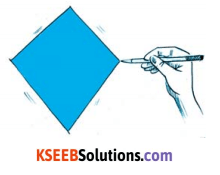# KSEEB Solutions for Class 6 Maths Chapter 4 Basic Geometrical Ideas Ex 4.5

Students can Download Chapter 4 Basic Geometrical Ideas Ex 4.5 Questions and Answers, Notes Pdf, KSEEB Solutions for Class 6 Maths helps you to revise the complete Karnataka State Board Syllabus and score more marks in your examinations.

## Karnataka State Syllabus Class 6 Maths Chapter 4 Basic Geometrical Ideas Ex 4.5

Question 1.
Draw a rough sketch of a quadrilateral PQRS. Draw its diagonals. Name them. Is the meeting point of the diagonals in the interior or exterior of the quadrilateral?Solution:Diagram are RR and QS, they meet at point O Which is the interior of & mn sq l PQRS.Question 2.
Draw a rough sketch of a quadrilateral KLMN. state.
a) Two pairs of opposite sides
$$\overline{AB}\$$, $$\overline{NM}\$$ and $$\overline{KN}\$$, $$\overline{ML}\$$
b) Two pairs of opposite angles
∠KLM and ∠KNM
∠LKM and ∠LMNc) Two pairs of adjacent sides
$$\overline{KL}\$$, $$\overline{KN}\$$ and $$\overline{NM}\$$, $$\overline{ML}\$$
$$\overline{KL}\$$, $$\overline{LM}\$$ and $$\overline{NM}\$$, $$\overline{NK}\$$d) Two pairs of adjacent angles.
∠K, ∠L, and ∠M, ∠N
∠K, ∠N, and ∠L, ∠M

error: Content is protected !!